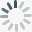×
BigML is working hard to support a wide range of browsers. Your experience will be better with:
Fusion Predictions
Jul2018One of the main goals of building a Fusion is to make predictions with it. Fusions can be used to predict categorical or numeric values. For classification problems, fusions average the per-class probabilities across all the component models. The class with the highest probability is predicted. For regression models, the final prediction is the result of averaging the per-model predicted values.

You can perform single predictions with fusions, if you want to predict just one instance; or batch predictions if you want to predict multiple instances at the same time. For single predictions you can ask for the prediction explanation, i.e., the per-field importances in the prediction. Learn more about fusions here!

predictions classification regression probabilities prediction explanation fusion
Fusion Evaluations
Jul2018Evaluate the performance of your Fusions to get an estimate of how good your model is at making predictions for new data. As with other supervised learning models, easily interpret the results with BigML evaluation visualizations such as the confusion matrix, the Precision-Recall, ROC, Gain and Lift curves for classification models. Since Fusion evaluations use the same performance metrics as other classification or regression models, you can quickly compare them with the BigML evaluation comparison tool. Learn more about fusions here!

evaluations classification regression supervised learning fusion
Fusions
Jul2018BigML is releasing Fusions, the new way to combine supervised models for improved performance. BigML Fusions combine multiple decision trees, ensembles, logistic regressions, and/or deepnets and aggregate their predictions to balance out the individual weaknesses of the single models for better performance and stability. Fusions are based on the assumption that the combination of multiple models often outperforms the component single models.

BigML provides a unique and intuitive visualization for Fusions: a Partial Dependence Plot for you to further inspect the Fusion results. Learn more about fusions here!

supervised classification regression dashboard partial dependence plot models logistic regression deepnets ensembles fusion
Sending Request...
Sending Request...